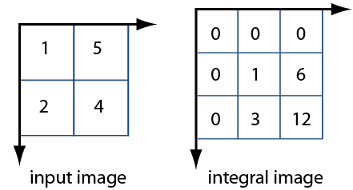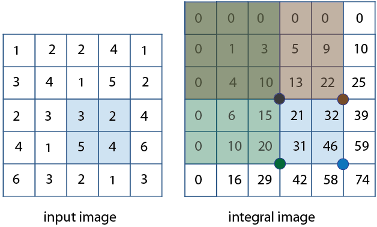## Integral Image

In an integral image, every pixel is the summation of the pixels above and to the left of it.

To illustrate, the following shows and image and its corresponding integral image. The integral image is padded to the left and the top to allow for the calculation. The pixel value at (2, 1) in the original image becomes the pixel value (3, 2) in the integral image after adding the pixel value above it (2+1) and to the left (3+0). Similarly, the pixel at (2, 2) in the original image with the value 4 becomes the pixel at (3, 3) in the integral image with the value 12 after adding the pixel value above it (4+5) and adding the pixel to the left of it (9+3).Using an integral image, you can rapidly calculate summations over image subregions. Integral images facilitate summation of pixels and can be performed in constant time, regardless of the neighborhood size. The following figure illustrates the summation of a subregion of an image, you can use the corresponding region of its integral image. For example, in the input image below, the summation of the shaded region becomes a simple calculation using four reference values of the rectangular region in the corresponding integral image. The calculation becomes, 46 – 22 – 20 + 10 = 14. The calculation subtracts the regions above and to the left of the shaded region. The area of overlap is added back to compensate for the double subtraction.In this way, you can calculate summations in rectangular regions rapidly, irrespective of the filter size. Use of integral images was popularized by the Viola-Jones algorithm. To see the full citation for this algorithm and learn how to create an integral image, see `integralImage`.